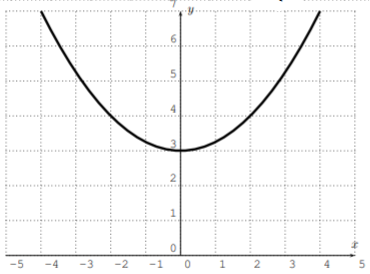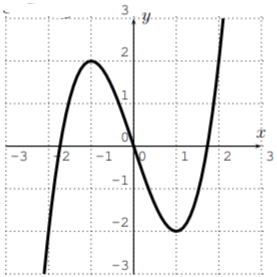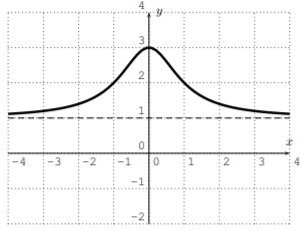# 5.3: Exercises

$$\newcommand{\vecs}{\overset { \rightharpoonup} {\mathbf{#1}} }$$ $$\newcommand{\vecd}{\overset{-\!-\!\rightharpoonup}{\vphantom{a}\smash {#1}}}$$$$\newcommand{\id}{\mathrm{id}}$$ $$\newcommand{\Span}{\mathrm{span}}$$ $$\newcommand{\kernel}{\mathrm{null}\,}$$ $$\newcommand{\range}{\mathrm{range}\,}$$ $$\newcommand{\RealPart}{\mathrm{Re}}$$ $$\newcommand{\ImaginaryPart}{\mathrm{Im}}$$ $$\newcommand{\Argument}{\mathrm{Arg}}$$ $$\newcommand{\norm}{\| #1 \|}$$ $$\newcommand{\inner}{\langle #1, #2 \rangle}$$ $$\newcommand{\Span}{\mathrm{span}}$$ $$\newcommand{\id}{\mathrm{id}}$$ $$\newcommand{\Span}{\mathrm{span}}$$ $$\newcommand{\kernel}{\mathrm{null}\,}$$ $$\newcommand{\range}{\mathrm{range}\,}$$ $$\newcommand{\RealPart}{\mathrm{Re}}$$ $$\newcommand{\ImaginaryPart}{\mathrm{Im}}$$ $$\newcommand{\Argument}{\mathrm{Arg}}$$ $$\newcommand{\norm}{\| #1 \|}$$ $$\newcommand{\inner}{\langle #1, #2 \rangle}$$ $$\newcommand{\Span}{\mathrm{span}}$$$$\newcommand{\AA}{\unicode[.8,0]{x212B}}$$

## Exercise $$\PageIndex{1}$$

Find a possible formula of the graph displayed below.

1.2.3.4.5.6.1. $$y=|x|+1$$
2. $$y=-\sqrt{x}$$
3. $$y=(x-2)^{2}+1$$
4. $$y=\dfrac{1}{x+2}+2$$
5. $$y=-(x+2)^{3}$$
6. $$y=-|x-3|+2$$

## Exercise $$\PageIndex{2}$$

Sketch the graph of the function, based on the basic graphs in Section 5.1 and the transformations described above. Confirm your answer by graphing the function with the calculator.

1. $$f(x)=|x|-3$$
2. $$f(x)=\dfrac 1 {x+2}$$
3. $$f(x)=-x^2$$
4. $$f(x)=(x-1)^3$$
5. $$f(x)=\sqrt{-x}$$
6. $$f(x)=4\cdot |x-3|$$
7. $$f(x)=-\sqrt{x}+1$$
8. $$f(x)=(\dfrac{1}{2}\cdot x)^2+3$$
1.2.3.4.5.6.7.8.## Exercise $$\PageIndex{3}$$

Consider the graph of $$f(x)=x^2-7x+1$$. Find the formula of the function that is given by performing the following transformations on the graph.

1. Shift the graph of $$f$$ down by $$4$$.
2. Shift the graph of $$f$$ to the left by $$3$$ units.
3. Reflect the graph of $$f$$ about the $$x$$-axis.
4. Reflect the graph of $$f$$ about the $$y$$-axis.
5. Stretch the graph of $$f$$ away from the $$y$$-axis by a factor $$3$$.
6. Compress the graph of $$f$$ towards the $$y$$-axis by a factor $$2$$.
1. $$y=x^{2}-7 x-3$$
2. $$y=(x+3)^{2}-7 \cdot(x+3)+1=x^{2}-x-11$$
3. $$y=-x^{2}+7 x-1$$
4. $$y=x^{2}+7 x+1$$
5. $$y=\dfrac{1}{9} x^{2}-\dfrac{7}{3} x+1$$
6. $$y=4 x^{2}-14 x+1$$

## Exercise $$\PageIndex{4}$$

How are the graphs of $$f$$ and $$g$$ related?

1. $$f(x)=\sqrt{x}, \quad g(x)=\sqrt{x-5}$$
2. $$f(x)=|x|, \quad g(x)=2 \cdot|x|$$
3. $$f(x)=(x+1)^3 , \quad g(x)=(x-3)^3$$
4. $$f(x)=x^2+3x+5, \quad g(x)=(2x)^2+3(2x)^2+5$$
5. $$f(x)=\dfrac 1 {x+3}, \quad g(x)=-\dfrac 1 x$$
6. $$f(x)= 2 \cdot |x|, \quad g(x)=|x+1|+1$$
1. shift to the right by $$5$$
2. stretched away from the $$x$$-axis by a factor $$2$$
3. shift to the right by $$4$$
4. compressed towards the $$y$$-axis by a factor $$2$$
5. shifted to the right by $$3$$ (to get the graph of $$y=\dfrac{1}{x}$$ ) and then reflected about the $$x$$-axis
6. compressed towards the $$x$$-axis by a factor $$2$$ (you get $$y=|x|$$) then shifted to the left by $$1$$ and up by $$1$$

## Exercise $$\PageIndex{5}$$

Determine, if the function is even, odd, or neither.

1. $$y=2x^3$$
2. $$y=5x^2$$
3. $$y=3x^4-4x^2+5$$
4. $$y=2x^3+5x^2$$
5. $$y=|x|$$
6. $$y=\dfrac{1}{x}$$
7.8.9.1. odd
2. even
3. even
4. neither
5. even
6. odd
7. even
8. neither
9. odd

## Exercise $$\PageIndex{6}$$

The graph of the function $$y=f(x)$$ is displayed below.Sketch the graph of the following functions.

1. $$y=f(x)+1$$
2. $$y=f(x-3)$$
3. $$y=-f(x)$$
4. $$y=2f(x)$$
5. $$y=f(2x)$$
6. $$y=f\left (\dfrac 1 2 x\right )$$
1.2.3.4.5.6.